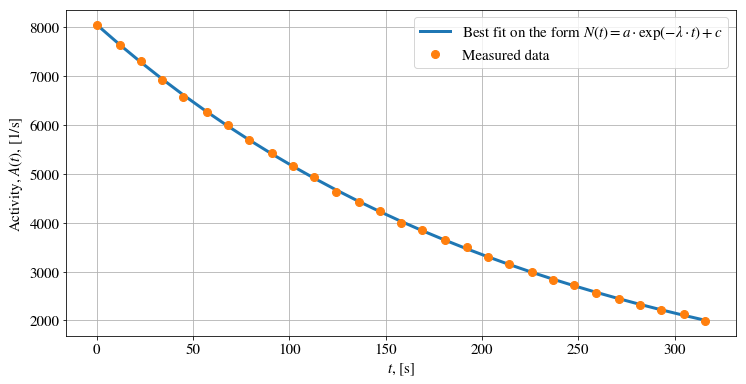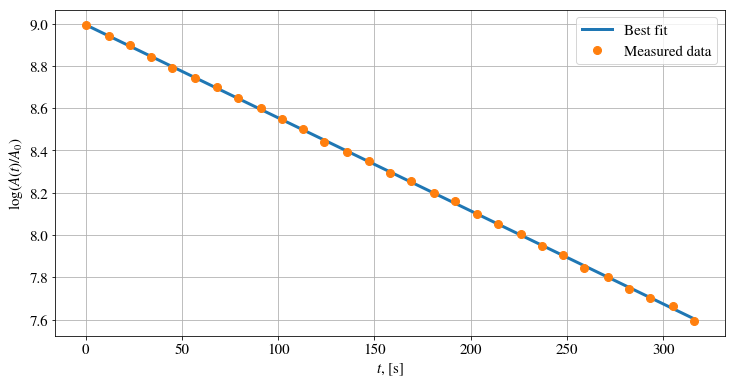Curve Fitting: Estimating the Half-Life of Ba-137m¶

Examples – Particle and Nuclear Physics¶

Last edited: January 21st 2019

Introduction¶

Cs-137, as well as a number of other radioactive isotopes, was released into the environment in almost all nuclear weapon tests and some nuclear accidents, most noticeably the Chernobyl disaster (1986) and the Fukushima Daiichi disaster (2011) . The parent isotope Cs-137, with a half-life of 30.1 years, beta decays into Ba-137m. This is a metastable state which further decays by 662 keV gamma emission, with a half-life of approximately 2.55 minutes, to the stable Ba-137 isotope .

In this notebook we will be using curve fitting to estimate the half life of the Ba-137m isotope. The main purpose of this notebook is to introduce a few curve fitting functions from SciPy and NumPy. The data is based on a set of measurements conducted in a labratory exercise at NTNU in 2016.

Radioactivity is a spontaneous process in which the nucleus of an unstable isotope looses energy by emitting radiation. This radiation includes alpha particles, beta particles (electron or positron), electron capture and gamma radiation.

The basic assumption of the statistical theory of radioactive decay is that the probability per unit time for the decay of an atom is constant, regardless of the age of the atom . That is,

\begin{equation} \lambda=-\frac{\text{d}N/\text{d}t}{N}, \end{equation}

where $N$ is the number of nuclei present at time $t$. $\lambda$ is often called the decay constant. Solving this differential equation yields the exponential law of radioactive decay,

\begin{equation} N(t)=N_0e^{-\lambda t}, \end{equation}

where $N_0=N(t=0)$. The half-life $t_{1/2}$ is the time taken for the activity of a given amount of a radioactive substance to decay to half of its initial value. Since the activity is proportional to the number of radioactive nuclei, the half-life becomes

\begin{equation} t_{1/2}=\frac{\ln(2)}{\lambda}. \end{equation}

The exponential law of radioactive decay does not take systematic errors and background radiation into count. A simple way to introduce this into our model is to superpose our equation on a constant background. That is,

\begin{equation} \tilde N(t)=N_0e^{-\lambda t} + c, \end{equation}

for some constant $c$.

A few notes on the measurements¶

A NaI-scintillator were used in the measurements. The NaI-scintillator crystal absorbs the gamma-photon emission produced in the Ba-137m decay and emits visible light proportional to the energy of the gamma photon. A photon multiplier tube absorbs the light photons and emits a voltage pulse proportional to the amount of visible light. The measurements consisted in finding the number of counts (every gamma-absorption event) in a given amount of time. Each measurement lasted for 10 seconds. Note that we are in fact measuring the activity $A(t)$ (number of counts per second), but the general formula above does not change.

The measured values are given in the following code snippet.

In :
# Number of counts in each measurement (10 seconds)
counts_Ba = [
80412., 76400., 73071., 69203., 65735., 62529., 59948., 56926.,
54243., 51490., 49255., 46256., 44152., 42339., 40003., 38525.,
36419., 35059., 33011., 31439., 29971., 28300., 27179., 25554.,
24475., 23143., 22187., 21279., 19845.
]
# Start time of measurement
times_Ba  = [
0.,    12.,    23.,    34.,    45.,    57.,    68.,    79.,
91.,  102.,   113.,   124.,   136.,   147.,   158.,   169.,
181.,   192.,  203.,   214.,   226.,   237.,   248.,   259.,
271.,   282.,  293.,   305.,   316.
]

Estimating the half life¶

In order to estimate the half life $\lambda_\text{Ba}$ of Ba-137m, we need to fit the exponential law of radioactivity to our data. We start by importing some needed packages, setting common figure parameters and defining a function for the exponential law of decay.

In :
import numpy as np
import matplotlib.pyplot as plt

# Set some figure parameters
newparams = {'figure.figsize': (12, 6), 'axes.grid': True,
'lines.markersize': 8, 'lines.linewidth': 3,
'font.size': 15, 'mathtext.fontset': 'stix',
'font.family': 'STIXGeneral'}
plt.rcParams.update(newparams)

def func(t, N0, lambd, c):
""" Callable model function to be used by the curve fitting
functions. It must take the independent variable as the
first argument and the parameters to fit as separate remaining
arguments.
"""
return N0 * np.exp(-lambd * np.array(t)) + c

curve_fit()¶

We will start by using scipy.optimize.curve_fit(), which uses non-linear least squares to fit an arbitrary function to data. The function has three input parameters: the model function (func), the measured $x$-data (times_Ba) and the measured $y$-data (counts_Ba). There are several optional arguments as well, but that is not a concern in this notebook. The function returns an array of the optimal values for the parameters (popt) and a corresponding covariance matrix (pcov). The diagonals of the covariance matrix provide the variance of the parameter estimates. The standard deviation is the square root of the variance.

In :
from scipy.optimize import curve_fit

Note that we we need to take the exponential of $\lambda\cdot t$, where $\lambda\approx 150\;\text{s}^{-1}$ and $t\leq 316\;\text{s}$. In other words, we need to calculate $\exp(150\cdot 316)=\exp(47400)\sim 10^{20585}$ numerically. This is a large number, and we will most likely get an overflow error. There are several ways to get around this, e.g. by taking the logarithm and perform a linear fit, or normalize the data. We will normalize such that the maximum time and maximum number of counts becomes unity.

In :
max_time = np.max(times_Ba)
max_counts = np.max(counts_Ba)

We are now ready to call curve_fit()!

In :
# Perform the curve fit on normalized data
popt, pcov = curve_fit(func, times_Ba/max_time, counts_Ba/max_counts)

# Standard deviation
perr = np.sqrt(np.diag(pcov))

# Parameter estimates reexpressed in ordinary units
lambd = popt/max_time
lambd_err = perr/max_time # Standard deviation in lambd
c = popt*max_counts
N0 = popt*max_counts

# Calculate half life with error
half_life = np.log(2)/lambd
half_life_err = half_life*lambd_err/lambd

print("Half life: %.1fs +- %.1fs"%(half_life, half_life_err))
Half life: 159.5s +- 2.0s

The estimated half-life of Ba-137m is $t_{1/2}=(159.5\pm 2.0)\;\text{s}$. The real half time of $153.12\;\text{s}$ is not in the error bounds. There are a lot of errors which might explain this inconsistency, for example the pureness of the source and error in the detection. Moreover, the data was measured during a short laboratory class.

Let's visualize the fit!

In :
plt.plot(times_Ba, func(times_Ba, N0, lambd, c)/10,
label=r'Best fit on the form $N(t)=a\cdot \exp(-\lambda\cdot t) + c$')
plt.plot(times_Ba, np.array(counts_Ba)/10, 'o', label='Measured data')
plt.xlabel(r'$t$, [s]')
plt.ylabel(r'Activity, $A(t)$, [1/s]')
plt.legend()
plt.show()polyfit()¶

Let's perform the same calculations as above using linear regression and numpy.polyfit(), which performs a least squares polynomial fit. The function has three input parameters: the measured $x$-data (times_Ba), the measured $y$-data (counts_Ba) and the degre of the polynomial (1). The function returns the polynomial coefficients, highest power first. In addition, we will use the optional argument cov=True, which tells the function to return the covariance matrix.

In :
from numpy import polyfit

By taking the logarithm on the exponential law of radioactive decay and let $c=0$, we obtain

\begin{equation} \log(\tilde N(t))=\log(N_0) -\lambda t. \end{equation}

We can thus perform a linear fit on the logarithm of our data!

In :
# Perform linear fit on the logarithm of the measurements
popt, pcov = np.polyfit(times_Ba, np.log(np.array(counts_Ba)/10), 1, cov=True)
perr = np.sqrt(np.diag(pcov))

# Calculate lambda with error
lambd = -popt
lambd_err = perr
# Calculate half life with error
half_life = np.log(2)/lambd
half_life_err = half_life*lambd_err/lambd
# Print results
print("Half life: %.1fs +- %.1fs"%(half_life, half_life_err))
Half life: 157.5s +- 0.4s

This result is about the same we obtained earlier. However, in this case we have not taken systematic errors (background) into count. Let's visualize the results.

In :
plt.plot(times_Ba, np.array(times_Ba)*popt[-2] + popt[-1],
label=r'Best fit')
plt.plot(times_Ba, np.log(np.array(counts_Ba)/10), 'o', label='Measured data')
plt.xlabel(r'$t$, [s]')
plt.ylabel(r'$\log(A(t)/A_0)$')
plt.legend()
plt.show()Further Work¶

• How can the half-life of an isotope, which decays into another unstable isotope, be estimated? (see e.g. Ref )